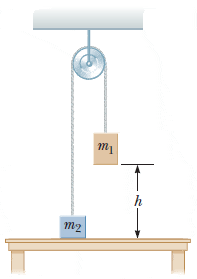# "Isolated system model" can someone explain this to me?

• doneky
In summary, the objects in this problem are treated separately because their energies and heights are different, making it easier to calculate their velocities and heights.

## Homework Statement

Two objects are connected by a light string passing over a light, frictionless pulley as shown in the figure below. The object of mass m1 = 4.80 kg is released from rest at a height h = 4.40 m above the table.(a) Using the isolated system model, determine the speed of the object of mass m2 = 3.00 kg just as the 4.80–kg object hits the table.
(b) Find the maximum height above the table to which the 3.00–kg object rises.

KE = 1/2*mv^2
PE = mgh

## The Attempt at a Solution

So, basically the answer is to combine the total changes in kinetic and potential energy, where nonconservative work equals zero.

But why? Why should these be treated separately instead of using a combined mass?

doneky said:
But why? Why should these be treated separately instead of using a combined mass?
I do not understand your question. You are to consider the combined energies, no? That effectively combines the masses.
An approach that does not directly combine the masses is to work in terms of forces, treating each mass separately. They become connected then by using common values for tension and acceleration.

The reason they are being treated as separate bodies is because the energy for each object is different from the other. If you were to treat both object as same, it would be very difficult to calculate the velocity of the connected bodies, because the velocity of m1 is different from m2. Same is the case is for GPE. The height from the reference point is different for both objects.